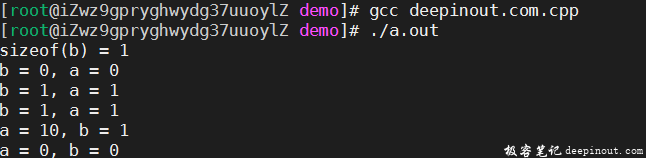# C++ 布尔类型(bool)

C++ 中的布尔类型：

• C++ 在C语言的基本类型系统之上增加了 bool，C语言用整型来代替，但浪费了空间
• C++ 中的bool 课取的值只有true和false
• 理论上 bool 只占用一个字节

true 代表真值， 编译器内部用1来表示

false代表非真值， 编译器内部用0来表示

## C++ 布尔类型的值

bool类型只有true（非0）和false（0）两个值。

C++编译器会将非0值转换为true，0值转换为false。

``````bool b = 0;
printf("b = %d \n", b);
b++;
printf("b = %d \n", b);
b = b - 3;
printf("b = %d \n", b);
``````

## 示例：布尔类型的使用

``````#include <stdio.h>

int main(int argc, char *argv[])
{
bool b = false;
int a = b;

printf("sizeof(b) = %d\n", sizeof(b));
printf("b = %d, a = %d\n", b, a);

b = 3;
a = b;

printf("b = %d, a = %d\n", b, a);

b = -5;
a = b;

printf("b = %d, a = %d\n", b, a);

a = 10;
b = a;

printf("a = %d, b = %d\n", a, b);

a = 0;
b = a;

printf("a = %d, b = %d\n", a, b);

return 0;
}
``````## 总结

• 可以定义 bool 类型的全局变量
• 可以定义 bool 类型的常量
• 可以定义 bool 类型的指针
• 可以定义 bool 类型的数组
• 。。。

• 微信订阅
• 回顶